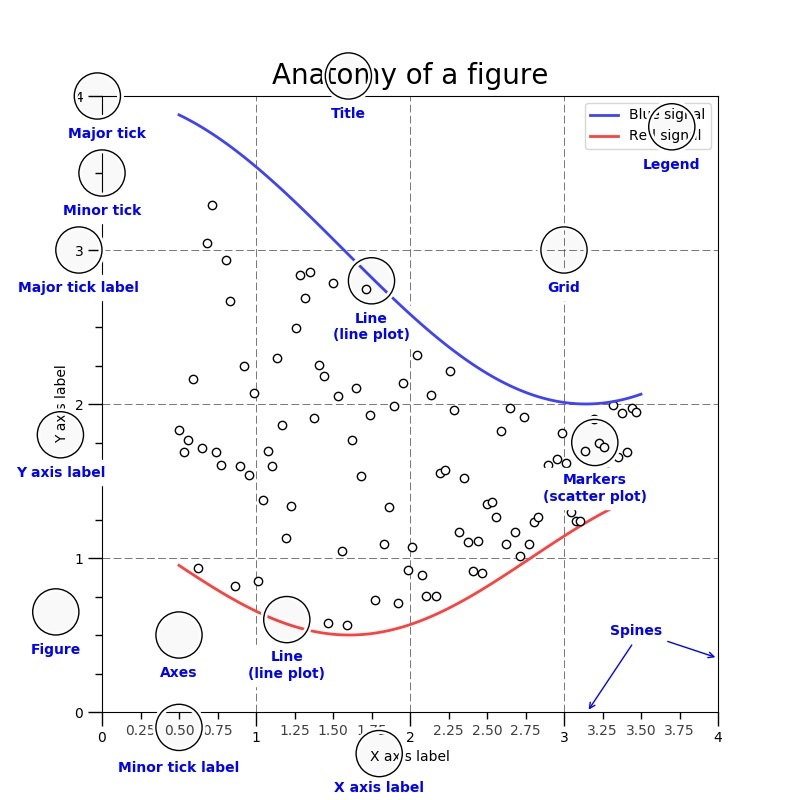# # ♢ Matplotlib

：Python 的第三方库，提供了一些模仿 MATLAB 的数学绘图函数，并基于 tkinter、qt5 等 GUI 库显示图像。

## # 折线图

• 使用绘图函数时，通常输入 numpy.array 等类型的数组，就会在坐标轴中绘制函数曲线。

• 可以用 `plt.plot()` 绘制一条折线，如下：

``````>>> import matplotlib.pyplot as plt
>>> x = [1, 2, 3]
>>> y = [-1, -2, -3]
>>> plt.plot(x, y)                                          # 绘制一条折线，x、y 数组分别表示各个点的 x 轴、y 轴坐标，两个数组的长度必须相同
[<matplotlib.lines.Line2D object at 0x000001F35773F820>]
>>> plt.plot(y, x, label='line2', linestyle='', marker='.') # 绘制第二条折线，它是散点线
[<matplotlib.lines.Line2D object at 0x000001F35801BB20>]
>>> plt.show()                                              # 显示 Figure 窗口
``````
• `plt.plot()` 的常用参数：

``````label='xx'          # 折线的名称
linewidth=1.0       # 线宽
linestyle='-'       # 线型
marker=''           # 节点标记的形状
color='#008000'     # 线的颜色。默认每次绘制时，会按蓝、橙、绿等顺序分配颜色
``````
• 线型有以下几种：
``````None
''
' '
'-'
'--'
'-.'
':'
'solid'     # 实线
'dashed'    # 虚线
'dotted'    # 点线
'dashdot'   # 点划线
``````
• 节点标记的形状有以下几种：
``````'s'         # 正方形
'o'         # 圆形
'^'         # 正三角形，分为四种朝向
'>'
'v'
'<'
'd'         # 正菱形
'p'         # 正五边形
'h'         # 正六边形
'8'         # 正八边形
``````

### # 动态折线图

``````import random

plt.ion()
last_dot = [0, 0]
for i in range(100):
new_dot = [i, random.randint(0, 10)]  # 生成当前点的坐标
# 每次循环会绘制一条新折线，与上一条折线连接，并采用统一的颜色
plt.plot([last_dot, new_dot], [last_dot, new_dot], color='blue')
last_dot = new_dot
plt.pause(0.1)

``````

## # Figure

• Matplotlib 提供了显示图像的 GUI 窗口，称为 Figure 。其结构如下：• 每个 Figure 上可以显示一个或多个坐标区（Axes）。
• 坐标区的坐标轴（Axis）有多种类型：
• xy 二维坐标轴
• xyz 三维坐标轴
• 极坐标轴
• 通常有两种绘图方式：

• 通过 `plt.plot()` 直接绘图，只能操作一个 Figure 实例。
• 通过 `plt.figure()` 创建 Figure 实例，以面向对象的方式绘图。

### # 交互模式

• 显示 Figure 窗口时默认采用非交互模式：

• 执行 `plt.plot()` 等函数时只会记录图像数据，不会实际绘制。执行 `plt.show()` 才会开始绘制图像，显示 Figure 。
• Figure 显示期间会一直阻塞前端，直到用户关闭其窗口。
• 在交互模式下：

• 执行 `plt.plot()``plt.show()` 等函数都会立即显示 Figure 窗口，如果已显示则更新显示。
• Figure 显示期间不会阻塞前端，从而可以继续执行其它代码来修改 Figure 。
``````>>> plt.ion()                     # 打开交互模式
>>> matplotlib.is_interactive()   # 判断是否处于交互模式
True
>>> plt.ioff()                    # 关闭交互模式
``````
• 执行 `plt.pause(n)` 会显示 Figure ，并阻塞前端 n 秒。

### # 关闭窗口

• 显示 Figure 窗口时，如果用户关闭该窗口：
• 非交互模式下，会自动销毁该 Figure 对象，导致不能再次显示。
• 交互模式下，可以再次显示。
• 可以在代码中主动关闭 Figure 窗口：
``````>>> plt.close()           # 关闭当前的 Figure
>>> plt.close(f1)         # 关闭指定的 Figure
>>> plt.close('test1')    # 关闭指定名称的 Figure（该名称不存在时并不会报错）
>>> plt.close('all')      # 关闭所有 Figure
``````

### # 排版

``````plt.axis('off')             # 不显示坐标轴（默认显示）
plt.axis([0, 10, 0, 1])     # 设置 x 轴和 y 轴的长度（如果设置了，显示时窗口比例会固定，否则会自动调整）

plt.title('Simple Plot')    # 设置图像的名称（默认为空），这并不是设置 Figure 窗口的 Title
plt.xlabel('x label')       # 设置 X 轴的名称（默认为空）
plt.ylabel('y label', fontsize=16))
plt.legend()                # 显示图例（这会自动提取每条折线的 label 名作为图例名）
plt.grid(True)              # 在坐标轴区域显示网格线

plt.cla()                   # 对当前 figure 中的所有坐标轴执行 axes.cla()，清除其显示内容
plt.clf()                   # 清除显示当前 figure 中的所有坐标轴

plt.savefig('./1.png')      # 保存图像
``````

### # 后端

• Matplotlib 将执行代码的线程称为前端，将显示 Figure 的线程称为后端。
• 管理 Matplotlib 后端的 GUI 引擎：
``````>>> matplotlib.get_backend()    # 查看当前引擎
'TkAgg'
>>> matplotlib.use('qt5agg')    # 使用指定的引擎
ImportError: Failed to import any qt binding
``````

### # 实例化

• 创建 Figure 实例：

``````>>> f1 = plt.figure()           # 创建一个 Figure
>>> f2 = plt.figure('test')
>>> f3 = plt.figure('test')
>>> f1.show()                   # 显示 Figure
>>> plt.show()                  # 显示所有已激活的 Figure
``````
• 相关 API ：

``````def figure(num=None,        # 设置 ID
figsize=None,    # 设置宽度、高度，比如 (3, 2.2)
dpi=None,        # 设置分辨率，比如 10 。实际的显示尺寸等于 figsize*dpi
facecolor=None,  # 设置背景色
edgecolor=None,  # 设置边框颜色
clear=False,     # 如果已存在相同 ID 的 Figure 则清除它
**kwargs
)
``````

创建 Figure 时，传入的 num 参数会用作其唯一 ID 。

• 如果没传入 num 参数，或者传入的 num 参数不是 int 类型，则会自动使用一个从 1 开始递增的 num 值。如下：
``````>>> f1.number
1
``````
• 如果传入的 num 参数是 str 类型，则会用作该 Figure 的 Title 。如下：
``````>>> f2.number
2
>>> f2.get_label()
'test'
``````
• 如果传入的 num 参数是 int 型，且已存在相同 ID 的 Figure ，则会激活该 Figure 并返回其引用。否则创建一个新的 Figure 并返回其引用。如下：
``````>>> f1 == f2
False
>>> f2 == f3
True
``````

### # Subplot

• 创建一个 Figure 时，默认只包含一个坐标轴图像，可以划分多个子图（Subplot）：

``````>>> fig, axes = plt.subplots(1)       # 创建一个 Figure ，采用 1 行的网格布局，每一格包含一个子图
>>> fig, axes = plt.subplots(2, 3)    # 创建一个 Figure ，采用 2 行、3 列的网格布局，每一格包含一个子图
>>> fig
<Figure size 640x480 with 4 Axes>
>>> axes                              # axes 数组中，每个元素对应一个子图的 axes
array([[<AxesSubplot:>, <AxesSubplot:>],
[<AxesSubplot:>, <AxesSubplot:>]], dtype=object)
>>> ax1 = axes[0, 0]                  # 选中第一个子图的 axes
>>> ax1.plot(x, x)
[<matplotlib.lines.Line2D object at 0x000001F35B72B1F0>]
>>> plt.show()
``````
• 可以给已有的 Figure 添加子图：

``````>>> f1 = plt.figure()
>>> f1.add_subplot(2, 3, 1)       # 让 Figure 采用 2 行、3 列的网格布局，将子图放在横向第 1 格内（只能位于第 1 行内）
<AxesSubplot:>
>>> f1.add_subplot(3, 3, (1,2))   # 让 Figure 采用 2 行、3 列的网格布局，将子图放在横向第 1 格至 第 2 格区域（只能位于第 1 行内）
<AxesSubplot:>
>>> f1.add_subplot(331)           # 当网格布局的行列数不超过 9 时，可以将前三个参数合并成一个三位整数
<AxesSubplot:>
``````
• 相关 API ：
``````def add_subplot(nrows=1, ncols=1, index=1, **kwargs)
``````
• index 的取值必须符合 `1 ≤ index ≤ nrows` ，否则会报错。
• 可以给同一个 Figure 多次添加子图，不过新的子图在显示时会覆盖旧的子图。

## # 显示图片

• 例：

``````>>> from matplotlib import pyplot as plt, image
>>> img = image.imread('./1.jpg')   # 读取本地图片
>>> p1 = plt.imshow(img)            # 将图片导入 Figure
>>> plt.show()                      # 显示 Figure
``````
• 读取到的图片对象实际上存储在 numpy 数组中：

``````>>> type(img)
<class 'numpy.ndarray'>
>>> img
array([[[ 72, 164, 201],
[ 64, 156, 193],
[ 66, 158, 195],
...,
[  0,  54,  74],
[  0,  53,  73],
[  0,  54,  74]]], dtype=uint8)
``````